# Mates y TIC - Maths and ICT

## Actividades de Matemáticas con TIC - Math Activities with ICT - - - (matesytic@gmail.com) Ricardo García Mesa

•# Archive for the '4º ESO'

## Trigonometric identities and homer measuring trees

Posted by ricardogm on 21st January 2019

Hi

We are going to prove these two famous trigonometic identities:

sin2(x) + cos2(x) = 1

tan(x)=cos(x)/sin(x)

that can be seen in this applet:​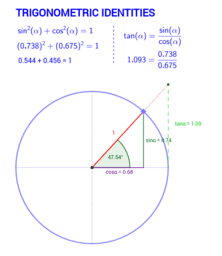And how to measure trees with trigonometry (we are going to do more or less the same thing):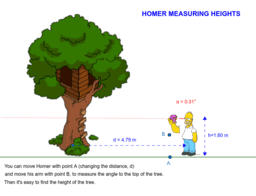Posted in 4º ESO | No Comments »

## Goniometric circumference

Posted by ricardogm on 18th January 2019Hi

Today we are going to create something similar to this: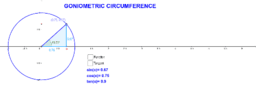The idea is to study the trigonometric ratios of angles greater than 90º.

Pay attention. Send me the file.

Now time to solve some problems in your notebook:

trigonometry-word-problems.pdfPosted in 4º ESO | No Comments »

## 3-gonometry-2

Posted by ricardogm on 10th January 2019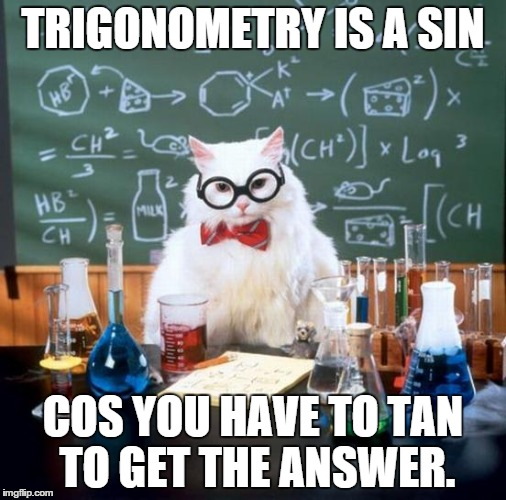Hi

We have some things to do:

0. Solve these problems in your notebook while I try to correct your homework. Make drawings, of course.

1 The known data for a right triangle ABC is a = 5 m and B = 41.7°. Solve the triangle.

2 The known data for a right triangle ABC is b = 3 m and B = 54.6°. Solve the triangle.

3 The known data for a right triangle ABC is a = 6 m and b = 4 m. Solve the triangle.

4 The known data for a right triangle ABC is b = 3 m and c = 5 m. Solve the triangle.

5 A tree 50 m tall casts a shadow 60 m long. Find the angle of elevation of the sun at that time.

6 An airship is flying at an altitude of 800 m when it spots a village in the distance with a depression angle of 12°. How far is the village from where the plane is flying over?

7 Find the radius of a circle knowing that a chord of 24.6 m has a corresponding arc of 70°.

8 Calculate the area of a triangular field, knowing that two of its sides measure 80 m and 130 m and between them is an angle of 70°.

9 Calculate the height of a tree, knowing that from a point on the ground the top of the tree can be seen at an angle of 30º and from 10 m closer the top can be seen at an angle of 60°.

10 The length of the side of a regular octagon is 12 m. Find the radii of the inscribed and circumscribed circles.

11 Calculate the length of the side and the apothem of a regular octagon inscribed in a circle with a radius of 49 centimeters.

12 Three towns A, B and C are connected by roads which form a triangle. The distance from A to C is 6 km and from B to C, 9 km. The angle between these roads is 120°. How far are the towns A and B from each other?

2. More problems.

The drawing below shows nicely what we are going to do:Posted in 4º ESO | No Comments »

## Tree-gonometry

Posted by ricardogm on 20th December 2018Hi

Today class has two parts:

a) Geogebra work. Pay attention and try to create a thing similar to the one the teacher is going to show you. Send it to me.

b) Game work. Try the games 2048 and Bloxorz and send me a review. Ciao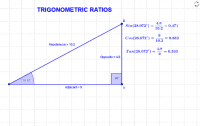Posted in 4º ESO | No Comments »

## Pruebas de diagnóstico 1

Posted by ricardogm on 13th December 2018Muy buenas

Hoy vamos a practicar con pruebas de diagnóstico de años anteriores:

Resolvéis en la libreta (sin copiar el enunciado) todas las que podáis.

Posted in 4º ESO | No Comments »

## Nonlinear systems of equations

Posted by ricardogm on 28th November 2018Hi

As you probably remember, last year we learnt to solve sytems of linear equations (first degree equations). Now we are going to try something a bit more difficult: systems of nolinear equations. In these systems, one or more of the equations are at least of second degree  and/or there is a product of variables in one of the equations.. The method we are going to use is substitution, because it’s the easiest, and in many cases the only practical way to solve these problems. Sometimes elimination works as well, or equalization. The graphic method is very helpful here, if you have access to geogebra, for instance. (HINT: I bet you have, it works on your phone! →Download page)

Here you can find some solved examples.

Here is a video showing one example. The audio is fine, but if you don’t have headphones the images are clear enough:

A written explanation is given in this book, page 31. Read it:

Book 4º ESO

Now some practice: Download these exercises and try to solve, in your notebook, as many as posible. The solutions are at the end, check them.

Another way to solve these or other systems is graphically. We are going to use Geogebra with the exercises. The teacher is going to show you how.Posted in 4º ESO | No Comments »

## Factoring polynomials

Posted by ricardogm on 22nd November 2018Hi

Now you have several tools to factorize polynomials (common factor, Ruffini, second degree formula).

For example, a second degree polynomial can be factorized using the formula, and writing it this way:

a x2 + bx + c =a · (x − s1) · (x − s2

being s1, s2, the solutions or roots of the polynomial.

An important word to use here is root. We say that x=a is a root or zero of the polynomial P(x) if P(a)=0. That means that this same x=a is a solution of the equation P(x)=0

With that in mind, you can use the method we saw for biquadratic equations as well. Use it to find the solutions (=roots) and then you can write the factorization this way:

ax4 + bx2 + c =a·(x-s1)(x-s2)(x-s3)(x-s4)

being s1, s2, s3, s4 the solutions or roots of the polynomial,Now some exercises to work out in your notebook. Use Symbolab to check them.

#### Factor and Calculate the Roots of the Following Polynomials

a) x3 + x2

b) 2x4 + 4x2

c) x2 − 4

d) x4 − 16

e)  9 + 6x + x2

f)g) x4 − 10x2 + 9

h) x4 − 2x2 − 3

i) 2x4 + x3 − 8x2 − x + 6

Now solve these equations. You should use the same tools, the only difference is the solution you have to give me.

a) 2x3 − 7x2 + 8x − 3

b) x3 − x2 − 4

c) x3 + 3x2 − 4 x − 12

d) 6x3 + 7x2 − 9x + 2

e) 3x5 − 18x3 + 27x =

f) 2x3 − 50x =

g) 2x5 − 32x =

h) 2x2 + x − 28 =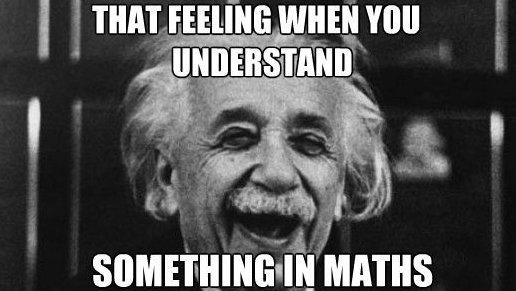Posted in 4º ESO | No Comments »

## The All Mighty Zero Product Property

Posted by ricardogm on 12th November 2018That’s it. The Zero Product Property simply states that if ab=0 , then either a=0  or  b=0 (or both). A product of factors is zero if and only if one or more of the factors is zero. Pretty logical, huh?

As simple as it seems, it’s so powerful that we can solve polynomial equations of any degree using this method,  if we can factor the polynomial (using Ruffini, for example).Here, we have found, for example, that 1 and -2 are solutions ofThere’s one solution more that we could find with Ruffini. Can you find it?

It also explains the relationship between factors of a polynomial and x-intercept of a function:

For example, if we plot this polynomial as a function:We get this:And you can see that the function crosses the x-axis on x=1 and x=-2 (and in x=-1, because we could also get this with ruffini)

All in all, it´s clear that zero is a very interesting number (although very feared by students all over the world).

A very complete (and lenghty) summary of this can be found here.

Some exercises to practice:

Exercises

And some more.Posted in 4º ESO | No Comments »

## Equations review

Posted by ricardogm on 8th November 2018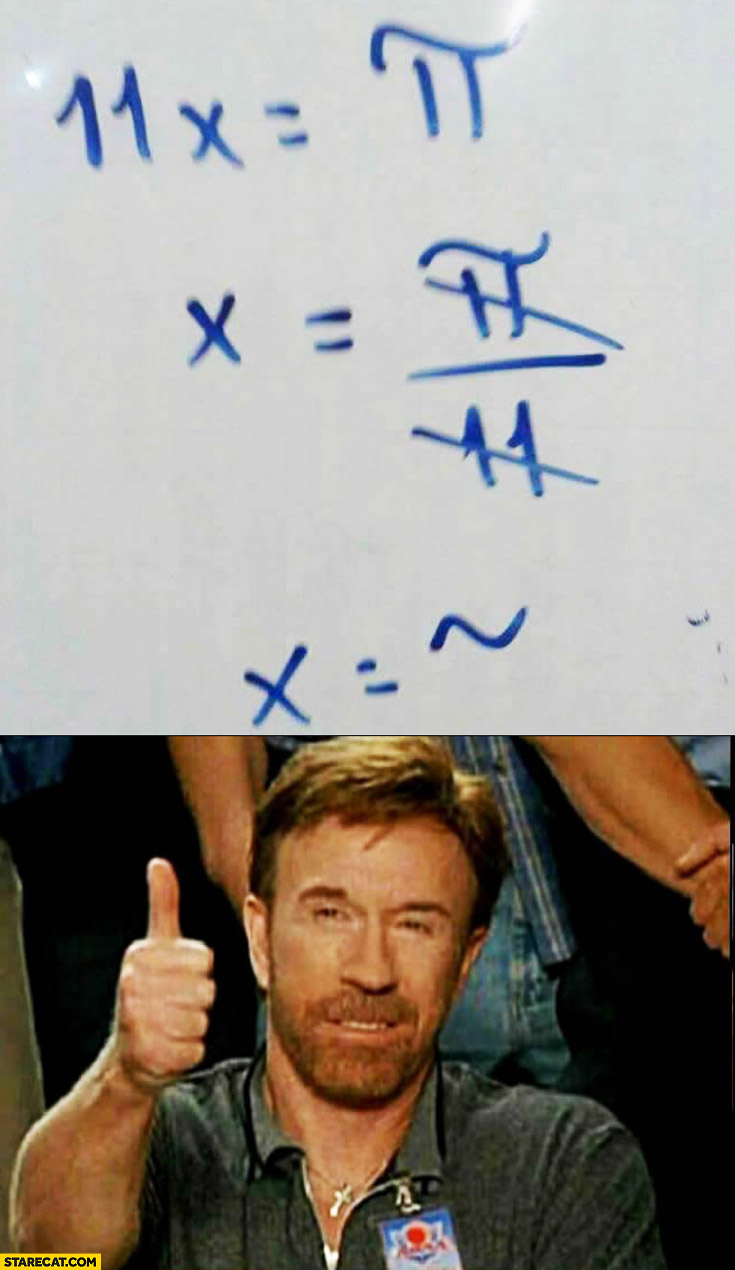Hi

1. First of all, 5 minutes to check the equations from yesterday using this page of Symbolab.

2. Now solve as many of these equations as you can in a blank sheet of paper (to give to the teacher at the end of the class, with your name on it) and check the solutions with Symbolab.

a) x4 − 10x2 + 9 = 0

b)c) x4 − 61x2 + 900 = 0

d)e)f)g) Two natural numbers differ by two units and the sum of their squares is 580. What are these numbers?

h) A rectangular garden 50 m long and 34 m wide is surrounded by a uniform dirt road. Find the width of the road if the total area of the garden and road is 540 m².Posted in 4º ESO | No Comments »

## Ruffini’s rule rules!

Posted by ricardogm on 25th October 2018Hi!

We are going to practice a little bit about division of polynomials, including Ruffini’s rule.

1. The teacher is going to show you some tools to correct these type of exercises. Pay attention. (NOTE: The programs use to show the answer in this format: D/d=Q+R/d)

2. Use these tools to correct the exercises from this week, at least the products and divisions.

3. Work out these divisions (in your notebook) using ruffini’s rule:5. Use the tools mentioned above to correct them.

6. Send me an email with the answers.

7. Now do the same thing with this exercise from your book: page 49, ex. 7.

8. Last thing to do: Find out who Paolo Ruffini was, and send me a few notes about him.Posted in 4º ESO | No Comments »